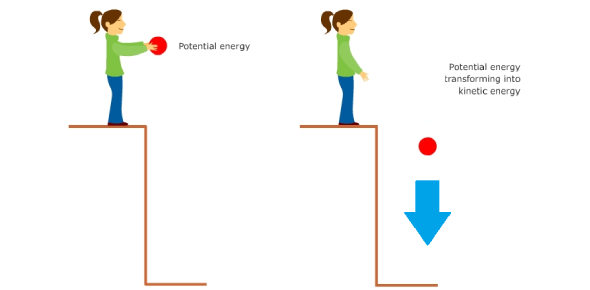# Physics Trivia Quiz: Gravitational Potential Energy And Kinetic Energy!

10 Questions | Total Attempts: 4375SettingsDo you know anything about gravitational potential energy and kinetic energy? This quiz will ascertain your knowledge of the topic. A ball being lifted above the ground it has gravitational potential energy, which is defined as the energy held by an object. If you are interested in the field of physics, this quiz is for you.

• 1.
What type of energy does the skater possess when he is at the greatest height on the track as shown in the picture above?
• A.

Elastic potential energy only

• B.

Gravitational potential energy only

• C.

Kinetic energy

• D.

Gravitational potential energy and kinetic energy

• 2.
What type of energy does the skater possess when he is moving pass the bottom of the track as shown in the picture above?
• A.

Elastic potential energy only

• B.

Gravitational potential energy only

• C.

Kinetic energy only

• D.

Gravitational potential energy and kinetic energy

• 3.
What type of energy does the skater possess as he is moving up the track as shown in the picture above?
• A.

Elastic potential energy only

• B.

Gravitational potential energy only

• C.

Kinetic energy only

• D.

Gravitational potential energy and kinetic energy

• 4.
Which of the following statements regarding the skater is correct as he moves from position A to B?
• A.

Skater possesses less gravitational potential energy at position A as compare to when he is at position B.

• B.

Skater possesses more energy at position A as compare to when he is at position B.

• C.

Skater possesses more gravitational potential energy at position A as compare to when he is at position B.

• D.

Skater possesses the same amount of gravitational potential energy at both positions A and B.

• 5.
Which of the following statements regarding the skater is correct as he moves from location A to B?
• A.

Skater possesses less kinetic energy at position A as compare to when he is at position B.

• B.

Skater possesses more energy at position A as compare to when he is at position B.

• C.

Skater possesses more kinetic energy at position A as compare to when he is at position B.

• D.

Skater possesses the same amount of kinetic energy at both positions A and B.

• 6.
The pie chart below shows the energy of the skater at a particular position as he moves through the track.Which of the following positions is the skater at?
• A.

Position A

• B.

Position B

• C.

Position C

• D.

Position D

• 7.
The picture above shows the skater at the starting point for his skate.  Which position in the picture indicates the greatest height the skater can reach.
• A.

Position A

• B.

Position B

• C.

Position C

• D.

Position D

• 8.
The skater moves from position A to D as shown in the picture below.The four bar graphs below show the energy of the skater at the above four positions but in the wrong order.Which of the following shows the correct order of the energy of the skater as he moves from position A to D?
• A.

I, III, IV, II

• B.

II, III, I, IV

• C.

II, IV, I, III

• D.

II, IV, III, I

• 9.
Which of these are examples of gravitational potential energy?
• A.

Ripe fruit before falling

• B.

Car parked at top of a hill

• C.

A yoyo before it is released

• D.

A man lying on the floor

• 10.
Gravity and weight are the same things.
• A.

True

• B.

False

Related TopicsBack to top